# RS Aggarwal Class 9 Solutions Chapter 11 - Circle

## RS Aggarwal Class 9 Chapter 11 - Circle Solutions Free PDF

A circle can be defined as a completely closed shape and the center of a circle is a point from where any kind of curve can be traced out and the distance from the center is always constant. Some of the different types of results which can be measured are the length of the circumference, Area of a circle, Equations and Tangent lines.

Some of the major terms that you will get to learn about this chapter are:

1. Arcs
2. Radius
3. Sector
4. Tangents
5. Chord
6. Diameter
7. Circumference
8. Segment
9. Center
10. Semicircle

## Download PDF of RS Aggarwal Class 9 Solutions Chapter 11 – Circle

The RS Aggarwal Class 9 solutions provided below will help students in exam preparation. All the answers provided in the solutions are easy and simple to understand. Scoring good marks will be easier for students if they practice from these solutions.

Exercise 11.1: Circle

Exercise 11.2: Circle

Exercise 11.3: Circle

Question 1: A chord of 16 cm is drawn in a circle of radius 10 cm. Find the distance of the chords the centre of the circle.

Sol.

Let AB be a chord of the given circle with center O and radius 10 cm. Then, OA = 10 cm and AB =  16 cm. From O, draw OL ⊥ AB. We know that the perpendicular from the center of a circle to a chord bisects the chord.

∴    $AL=\frac{1}{2}\times AB$

= $=\left ( \frac{1}{2}\times16 \right )$ cm = 8cm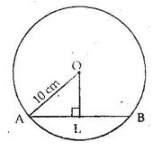From rt angled Δ OLA, we have

OA ² = OL ² + AL ²

⇒ OL ² = OA ² – AL ²

= 10 ² – 8 ²

= 100 – 64 = 36

$OL=\sqrt{36}$ = 6 cm

Question 2:  Find the length of a chord which is at a distance of 3 cm from the centre of a circle of radius 5 cm.

Sol.

Let AB be the chord of the given circle with centre O and radius 5 cm.

From O, draw OL ⊥ AB

Then, OA = 5 cm and OL = 3 cm   [given]

We know that the perpendicular from the centre of a circle to a chord bisects the chord.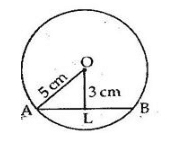Now, in rt  angled Δ OLA, we have

OA ² = OL ² + AL ²

⇒ AL ² = OA ² – OL ²

⇒ AL ² = 5² – 3²

= 25 – 9 = 16

∴               $AL=\sqrt{16}$ = 4cm

So, AB = 2 AL

=  (2 × 4)cm = 8 cm

∴    The length of the chord is 8 cm.

Question 3:  A chord of length 30 cm is drawn at a distance of 8 cm from the centre of the circle. Find out the radius of the circle.

Sol.

Let AB be the chord of the given circle with center O. Draw OL ⊥ AB.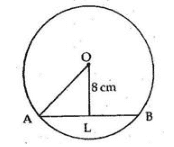Then, OL is the distance from the center to the chord.

So, we have AB = 30 cm and OL = 8 cm

We know that the perpendicular from the centre of a circle to a chord bisects the chord.

$AL = \frac{1}{2}\times AB$

= $=\left ( \frac{1}{2}\times 30 \right )$ cm = 15 cm

Now, in rt  angled Δ OLA, we have

OA ² = OL ² + AL ²

= 8² + 15²

= 64 + 225 = 289

$OA=\sqrt{289}$ = 17 cm

∴ The radius of the circle is = 17 cm.

Question 4:  In a circle of radius 5 cm, AB and CD are two parallel chords of length 8 cm and 6 CM respectively. Calculate the distance between the chords if they are

1. On the same side of the centre .
2. On the opposite side of the centre.

Sol.

(i) Let AB and CD be two chords of a circle such that AB || CD which is on the same side of the circle. Also AB = 8 cm and CD = 6 cm, OB = OD = 5 cm. Join OL and LM.

Since the perpendicular from the centre of a circle to a chord bisects the chord.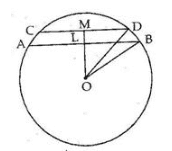We have $LB=\frac{1}{2}\times AB$

= $=\left ( \frac{1}{2}\times 8 \right )$ cm = 4 cm

And $MD=\frac{1}{2}\times CD$

= $=\left ( \frac{1}{2}\times 6 \right )$ cm = 3 cm

Now, in rt  angled Δ BLO, we have

OB² = LB² + LO²

⇒ LO² = OB² – LB²

= 5² – 4²

= 25 – 16 = 9

$LO=\sqrt{9}$ = 3 cm

Again in rt  angled Δ DMO, we have

OD²  = MD²  + MO²

MO²  = OD²  – MD²

= 5²  – 3²

= 25 – 9 =16

$MO=\sqrt{16}$ = 4 cm

∴ The distance between the chords = (4 – 3) cm = 1 cm.

(ii) Let AB and CD be two chords of a circle such that AB || CD and they are on the oppsite side of the center. AB = 8 cm and CD = 6 cm. Draw OL ⊥ AB and OM ⊥ CD.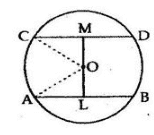Join OA and OC

Then OA = OC + 5 cm (radius)

Since the perpendicular from the centre of a circle to a chord bisects the chord.

We have

$AL=\frac{1}{2}AB$

= $\left ( \frac{1}{2}\times 8 \right)$ cm = 4 cm.

Also $CM=\frac{1}{2}CD$

= $\left ( \frac{1}{2}\times 6 \right)$ cm = 3 cm.

Now, in rt  angled Δ OLA, we have

OA ² = OL ² + AL ²

⇒ OL ² = OA ² – AL ²

= 5² – 4²

= 25 – 16 = 9 cm

∴               $OL=\sqrt{9}$ = 3cm

Again in rt angled Δ OMC, we have

OC² = OM² + CM²

⇒ OM² = OC² – CM²

= 5² – 3²

= 25 – 9 = 16

⇒               $OM=\sqrt{16}$ = 4cm

∴ The distance between the chords = (4 + 3) cm =

Question 5: In a given figure , the diameter CD of a circle with Centre O is perpendicular to chord Ab . If AB= 12cm and CE= 3 cm .Calculate the radius of the circle.

Sol.

CD is the diameter of a circle with center O, and is perpendicular to chord AB, Join OA.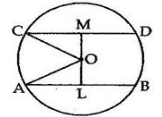AB = 12 cm and CE = 3 cm

Let OA = OC = r cm

Then, OE = (r – 3) cm

Since the perpendicular from the centre of a circle to a chord bisects the chord.

We have

$AE=\frac{1}{2}AB$

= $\left ( \frac{1}{2}\times 12 \right)$ cm =6 cm

Now, in rt  angled Δ OEA, we have

OA ² = OE ² + AE ²

⇒ r² = (r – 3)² + 6²

⇒     = r² -6r + 9 +36

⇒ r² – r² + 6r = 45

⇒   6r =45

⇒    $r=\frac{45}{6}$ = 7.5 cm

∴ OA, the radius of the circle is 7.5 cm.

Question 6: In the given figure, a circle with Centre O is given in which a diameter AB bisects the chord CD at a point E  such that CE=ED= 8 cm and EB= 4 cm. Find the radius of the circle.

Sol.

AB is the diameter of a circle with center O which bisects the chord CD at point E.

CE = ED = 8 cm and EB = 4 cm, Join OC.

Let OC = OB = r cm.

Then,

OE = (r – 4) cm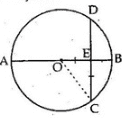Now, in rt angled Δ OEC, we have

OC ² = OE ² + EC ²

⇒ r² = (r – 4)² + 8²

⇒ r² = r² -8r + 16 +64

⇒ r² – r² + 8r = 80

⇒   8r =80

⇒    $r=\frac{80}{8}$ = 10 cm

∴ OE, the radius of the circle is 10 cm.

Question 7: in the adjoining figure OD is perpendicular to chord AB of a circle with Centre O. If BC is a diameter, show that AC || OD and AC = 2 × OD

Sol.

Given: OD ⊥ AB of a circle with center O, BC is a diameter.

To Prove: AC || OD and AC = 2 × OD

Construction: Join AC.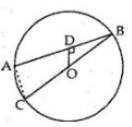Proof:

We know that the perpendicular from the centre of a circle to a chord bisects the chord.

Here OD ⊥ AB

⇒ D is the mid–point of AB

⇒ AD = BD

Also, O is the mid–point of BC

OC = OB

Now, in Δ ABC, D is the mid – point of AB and O is the mid–point of BC.

Midpoint Theorem: The line segment joining the midpoints of any two sides of a triangle is parallel to the third side and equal to half of it.

∴ OD || AC and $OD = \frac{1}{2} AC$

∴        AC = 2 × OD

Question 8: In the given figure, O is the centre of a circle in which chord AB and CD intersect at PO  bisect ∠BPD . Prove that AB = CD .

Sol.

Given: O is the center in which chords AB and CD intersect at P such that bisects ∠BPD

To Prove:    AB = CD

Construction: Draw OE ⊥ AB and OF ⊥ CD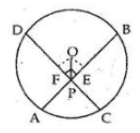Proof:

In Δ OEP and Δ OFP

∠ OEP = ∠ OFP [Each equal to 90°]

OP = OP [common]

∠ OPE = ∠ OPF [Since OP bisects ∠ BPD]

Thus, by Angle-Side-Angle criterion of congruence, we have

Δ OEP ≅ Δ OFP [By ASA]

The corresponding the parts of the congruent triangles are equal

⇒ OE = OF [CP.CT]

⇒ Chords AB and CD are equidistant from the center O.

⇒ AB = CD [∵ chords equidistant from the center are equal]

∴ AB = CD

Question 9: Prove that the diameter of the circle perpendicular to one of the two parallel chords of a circle is perpendicular to the other and bisect it.

Sol.

Given: AB and CD are two parallel chords of a circle with center O. POQ is a diameter which is perpendicular to AB.

To Prove: PF ⊥ CD and CF = FD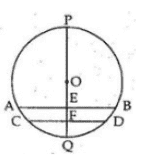Proof:

AB || CD and POQ is a diameter.

∠ PEB = 90° [Given]

Then, ∠ PFD = ∠ PEB [AB || CD, Corresponding angles]

Thus, PF ⊥ CD

So, OF ⊥ CD

We know that the perpendicular from the center of a circle to chord, bisect the chord.

∴ CF = FD.

Question 10: Prove that two different cycles cannot intersect each other at more than two points.

Sol.

If possible let two different circles intersect at three distinct point A, B, and C.

Then, these points are noncollinear. Do unique circles can be drawn to pass through these points. This is a contradiction.

Question 11: Two circle of radius 10 cm and 8 cm intersect each other, and the length of the common chord is 12 Cm. Find the distance between the centres.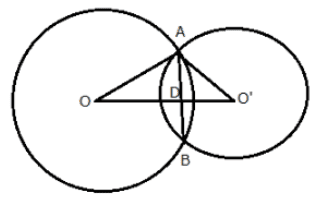Sol.

OA = 10 cm    and    AB = 12 cm

∴                 $AD=\frac{1}{2}AB$

= $\left ( \frac{1}{2}\times 12 \right)$ cm =6 cm

Now, in rt angled Δ ADO, we have

OA ² = AD ² + OD ²

⇒ OD ² = OA ² – AD ²

= 10² – 6²

= 100 – 36 = 64

$OD=\sqrt{64}$ = 8 cm

Again, we have O’A = 8 cm

In rt angled Δ ADO’, we have

O’A ² = AD ² + O’D ²

⇒ O’D ² = O’A ² – AD ²

= 8² – 6²

= 64 – 36 = 28

$OD=\sqrt{28}$ = $2\sqrt{7}$ cm

∴                      O’O = (OD + O’D)

= $(8+2\sqrt{7})$ cm

∴ The distance between their centers is $(8+2\sqrt{7})$ cm

### RS Aggarwal Class 9 Solutions Chapter 11 – Circle

Class 9 students should start their exam preperation by solving the RS Aggarwal Maths solutions. With the help of these solutions, students can clear their concepts and can also solve different types of questions that might be asked in the final exam. It teaches different methods to solve tough questions in a simple way. It is one of the valuable reference material for revision purpose as it covers the entire syllabus.